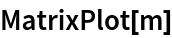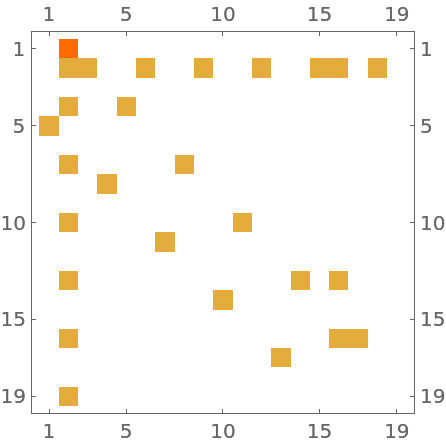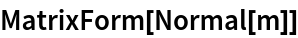Function Repository Resource:

Find the adjacency matrix for vertices in a hypergraph

Contributed by: Stephen Wolfram

## Details and Options

The entries in the hypergraph adjacency matrix count the number of cooccurrences of vertices within edges.
A hypergraph is represented by a list of edge lists, with each edge list containing a list of vertices in that edge.
ResourceFunction["HypergraphAdjacencyMatrix"] returns a SparseArray object, which can be converted to an ordinary matrix using Normal.

## Examples

### Basic Examples (3)

Make an adjacency matrix from a fairly simple hypergraph:

 In:=Out=Use MatrixPlot to visualize the matrix:

 In:=Out=In:=Out=